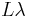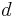# Difference between revisions of "IKONOS"

IKONOS is a commercial earth observation satellite. Details about the sensor in Digital Globe's IKONOS Data Sheet

## Pre-Processing Overview

Tyipically, multispectral data are converted into Reflectance before they are subjected in multispectral analysis techniques (image interpretation, band arithmetic, vegetation indices, matrix transformations, etc.). As with remotely sensed data acquired by other sensors, IKONOS raw image digital numbers (DNs) can be converted to physical quantities such as at-sensor Radiance or Reflectance. The latter can be differentiated in Top of Atmosphere Reflectance (ToAR) which does not account for atmospheric effects (absorption or scattering) and in Top of Canopy Reflectance (ToCR) which introduces a "correction" for atmospheric effects.

Converting to Top of Atmosphere Reflectance, also referred to as Planetary Reflectance, can be done by using the following equation:

Failed to parse (lexing error): \rho_p = \pi * L\lambda * d^2 / ESUN\lambda * cos(θ_S)

where:

•$\rho$ - Unitless Planetary Reflectance
•$\pi$ - mathematical constant (3.14159265358)
•$L\lambda$ spectral Radiance at the sensor's aperture, from equation... ToADD
•$d$ - Earth-Sun distance in astronomical units, interpolated values
•$Esun$ - Mean solar exoatmospheric irradiance(s) (W/m2/μm), interpolated values
• Failed to parse (lexing error): cos(θ_s) - Solar zenith angle, from the image acquisition's metadata